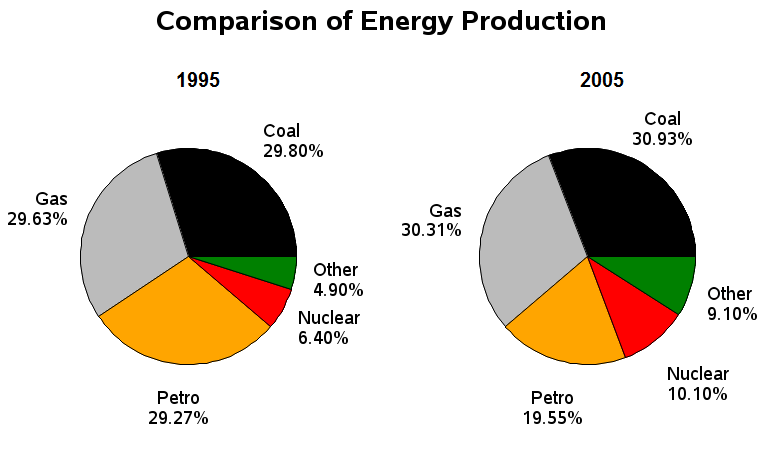Uncategorized

# Pie Charts

Before you start to explain a pie chart you should have the following concepts:-

• a title
• a key
• each piece of a pie chart labelled
• a unit of measurement
##### ExampleLook at the graph above, we can see that

Therefore we can say that:-

• a title= Comparision of energy production
• Date= 1995 and 2005
• a key= no key
• each piece of a pie chart labelled= other, nuclear, Petro, gas, coal
• a unit of measurement= Percentage

#### Understanding the pie chart

Hence, it can be understood that in 1995, the highest production of energy was by the use of coal i.e. 29.8%.